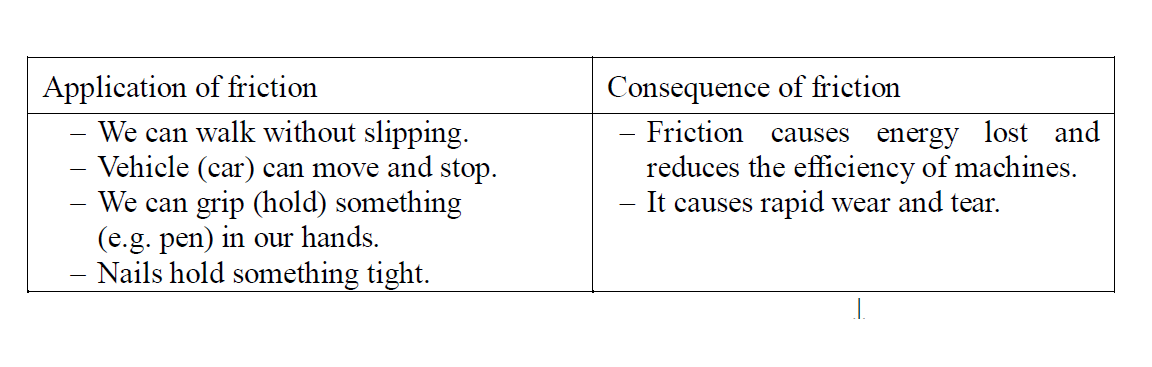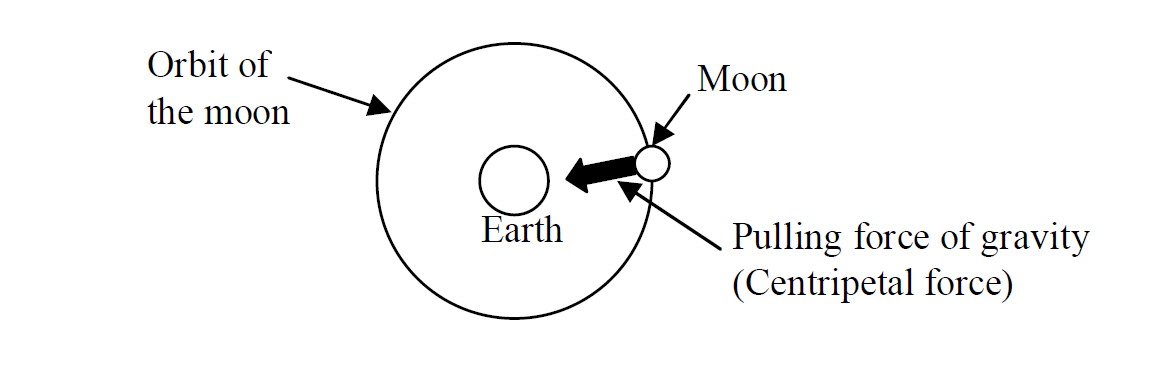# Forces

Force is refered to has a pull or a push.

Ability of force:

- Force can change the size or shape of a body.

- Force can change the motion of a body.

The unit of force is Newton [N] and It is a vector

E.G Weight is a kind of force.

##### Instrument for Measuring force

spring balance is used to measure Force , as weight is measured.

#### Resultant force

If two forces or more act on an object, they can be simplified. It is called a resultant force.

Note that If a resultant force is 0N on an object, then the forces are balanced on the object.

#### Newton’s first law of motion

Law If all the forces are balanced on a body,

- if it is at rest, it will continue to stay at rest

- if it is moving, it will keep moving at a constant speed in a straight line.

The property of a body that resists a change to its motion is called Inertia. Inertia depends on the mass of an object. If something has a high resistance to change of motion (a high mass), it is said to have high inertia.

#### Example

Which is easier to move, a wire car or a truck?

Solution

Answer: The wire car is easier to move than the truck because the wire car has lower inertia. (The truck has higher inertia.)

#### Newton’s second law of motion

Law Unbalanced forces acting on a body produce acceleration in the direction of the force

This acceleration is directly proportional to the force and inversely proportional to the mass of the body.

Formula for Force

$F=ma$

F: Force (Resultant force) [N]

m: mass [kg]

a: acceleration [m/s2]

#### Newton’s third Law of motion

For every force exerted on an object an equal and opposite force acts on that object.

#### Example

A force of 350N acts on an object of mass 10kg. Find the acceleration.

Solution

$F=ma$

Given that

m = 10kg

a = ?

F = 350N

$\mathrm{350}=10a$

$\frac{\mathrm{10a}}{10}=\frac{\mathrm{350}}{10}$

Answer: $a={\mathrm{35m/s}}^{2}$

#### Example 2

A man pushes an 8kg luggage on the smooth floor. It starts from rest and reaches 15m/s in 5s.

(a) What is its acceleration?

(b) What is the acting force on the luggage?

Solutions

(a)

$a=\frac{\mathrm{V - U}}{t}$

v = 15m/s

u = 0m/s

t = 5s

a = ?

$a=\frac{\mathrm{15 - 0}}{5}$

$a=\frac{\mathrm{15}}{5}$

$a={\mathrm{3m/s}}^{2}$

(b)

$F=ma$

m = 8kg

$a={\mathrm{3m/s}}^{2}$

F = ?

$F=8\left(3\right)$

Answer: $F=24N$

#### Friction

Friction is a force which acts to stop the motion of two touching surfaces.

- Friction acts in the opposite direction of the motion or tendency of motion.

- The larger friction exists between rougher surfaces.

For example, if you push a luggage, friction is caused between the luggage and the floor. Its direction is opposite to pushing force and it resists moving. If you push it on a smooth floor like ice, friction reduces and it is easy to push.

##### Application of frictionTo reduce friction, lubricants (oil, grease) are added to machines.

#### Centripetal force

The force of circular motion is always at right angle to the motion. The direction of the force is always towards the centre of circular motion. This force is called the centripetal force.The moon is in a circular orbit round the earth because the earth pulls the moon by the force of gravity.